# Tropics and polar zones

What percentage of the Earth’s surface lies in the tropical, temperate and polar zone?
Individual zones are bordered by tropics 23°27' and polar circles 66°33'

Result

p1 =  39.795 %
p2 =  51.946 %
p3 =  8.259 %

#### Solution:

$T=23 + 27/60=\dfrac{ 469 }{ 20 }=23.45 \ ^\circ \ \\ P=66 + 33/60=\dfrac{ 1331 }{ 20 }=66.55 \ ^\circ \ \\ \ \\ r=1 \ \\ \cos (90-P)=x_{1} : r \ \\ x_{1}=r \cdot \ \cos( ( 90-P) ^\circ \rightarrow\ \text{rad})=r \cdot \ \cos( ( 90-P )^\circ \cdot \ \dfrac{ \pi }{ 180 } \ )=1 \cdot \ \cos( ( 90-66.55 )^\circ \cdot \ \dfrac{ 3.1415926 }{ 180 } \ )=0.91741 \ \\ h_{1}=r - x_{1}=1 - 0.9174 \doteq 0.0826 \ \\ \ \\ \cos (90-T)=x_{2} : r \ \\ x_{2}=r \cdot \ \cos( ( 90-T) ^\circ \rightarrow\ \text{rad})=r \cdot \ \cos( ( 90-T )^\circ \cdot \ \dfrac{ \pi }{ 180 } \ )=1 \cdot \ \cos( ( 90-23.45 )^\circ \cdot \ \dfrac{ 3.1415926 }{ 180 } \ )=0.39795 \ \\ h_{2}=r - x_{2}=1 - 0.3979 \doteq 0.6021 \ \\ \ \\ S_{1}=2 \pi \cdot \ r \cdot \ h_{1}=2 \cdot \ 3.1416 \cdot \ 1 \cdot \ 0.0826 \doteq 0.5189 \ \\ S_{2}=2 \pi \cdot \ r \cdot \ h_{2}=2 \cdot \ 3.1416 \cdot \ 1 \cdot \ 0.6021 \doteq 3.7828 \ \\ \ \\ S_{3}=2 \pi \cdot \ r^2=2 \cdot \ 3.1416 \cdot \ 1^2 \doteq 6.2832 \ \\ \ \\ p_{1}=100 \cdot \ \dfrac{ S_{3}-S_{2} }{ S_{3} }=100 \cdot \ \dfrac{ 6.2832-3.7828 }{ 6.2832 } \doteq 39.7949 \doteq 39.795 \%$
$p_{2}=100 \cdot \ \dfrac{ S_{2}-S_{1} }{ S_{3} }=100 \cdot \ \dfrac{ 3.7828-0.5189 }{ 6.2832 } \doteq 51.9459 \doteq 51.946 \%$
$p_{3}=100 \cdot \ \dfrac{ S_{1} }{ S_{3} }=100 \cdot \ \dfrac{ 0.5189 }{ 6.2832 } \doteq 8.2592 \doteq 8.259 \%$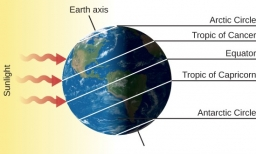Our examples were largely sent or created by pupils and students themselves. Therefore, we would be pleased if you could send us any errors you found, spelling mistakes, or rephasing the example. Thank you!

Leave us a comment of this math problem and its solution (i.e. if it is still somewhat unclear...):Be the first to comment!Tips to related online calculators

## Next similar math problems:

1. What percentageWhat percentage of the Earth’s surface is seen by an astronaut from a height of h = 350 km. Take the Earth as a sphere with the radius R = 6370 km
2. Altitude difference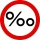What a climb in per mille of the hill long 4 km and the altitude difference is 6 meters?
3. The bus stop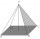The bus stop waiting room has the shape of a regular quadrilateral pyramid 4 m high with a 5 m base edge. Calculate how many m2 roofing is required to cover the three walls of the sheathing, taking into account 40% of the additional coverage.
4. The spacecraft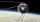The spacecraft spotted a radar device at altitude angle alpha = 34 degrees 37 minutes and had a distance of u = 615km from Earth's observation point. Calculate the distance d of the spacecraft from Earth at the moment of observation. Earth is considered
5. Triangular prism,The regular triangular prism, whose edges are identical, has a surface of 2514 cm ^ 2 (square). Find the volume of this body in cm3 (l).
6. Top of the towerThe top of the tower has the shape of a regular hexagonal pyramid. The base edge has a length of 1.2 m, the pyramid height is 1.6 m. How many square meters of sheet metal is needed to cover the top of the tower if 15% extra sheet metal is needed for joint
7. Right angleIf a, b and c are two sides of a triangle ABC, a right angle in A, find the value on each missing side. If b=10, c=6
8. Angle of the body diagonalsUsing vector dot product calculate the angle of the body diagonals of the cube.
9. Five-gon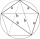Calculate the side a, the circumference and the area of the regular 5-angle if Rop = 6cm.
10. AnnulusTwo concentric circles with radii 1 and 9 surround the annular circle. This ring is inscribed with n circles that do not overlap. Determine the highest possible value of n.
11. Coordinates of a centroindLet’s A = [3, 2, 0], B = [1, -2, 4] and C = [1, 1, 1] be 3 points in space. Calculate the coordinates of the centroid of △ABC (the intersection of the medians).
12. Tetrahedral pyramid 8Let’s all side edges of the tetrahedral pyramid ABCDV be equally long and its base let’s be a rectangle. Determine its volume if you know the deviations A=40° B=70° of the planes of adjacent sidewalls and the plane of the base and the height h=16 of the p
13. Power line poleFrom point A, the power line pole is seen at an angle of 18 degrees. From point B to which we get when going from point A 30m away from the column at an angle of 10 degrees. Find the height of the power pole.
14. Free space in the gardenThe grandfather's free space in the garden was in the shape of a rectangular triangle with 5 meters and 12 meters in length. He decided to divide it into two parts and the height of the hypotenuse. For the smaller part creates a rock garden, for the large
15. Steps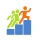Find the height between the two floors if you know that the number of steps between the two floors is 18, the gradient is 30º and the length of the step is 28.6 cm. Report the result in centimeters to the nearest centimeter.
16. PerimetersA rectangle has a perimeter of 16p centimeters, it had a width of 2p centimeters. Each side of an equilateral triangle is 1/2 the length of the rectangle. Find the total perimeter of the rectangle and the triangle if p=8.
17. A cell tower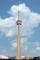A cell tower is located at coordinates (-5, -7) and has a circular range of 12 units. If Mr. XYZ is located at coordinates (4,5), will he be able to get a signal?